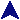Scalar VariablesCode Examples```# Various operations on scalar (string) variables.
\$fred = "Fred here";
\$barney = 56;
\$sum = 10 + \$barney;
print 'The variable \$fred' . " contains \$fred.\n";
print "Sum is \$sum.\n";

```